### 重要方法

• 获取类的方法 `foName`
• 实例化类对象的方法 `newInstance`
• 获取函数的方法 `getMethod`
• 执行函数的方法 `invoke`
• 获得构造方法 `getConstructor`

#### Runtime类的分析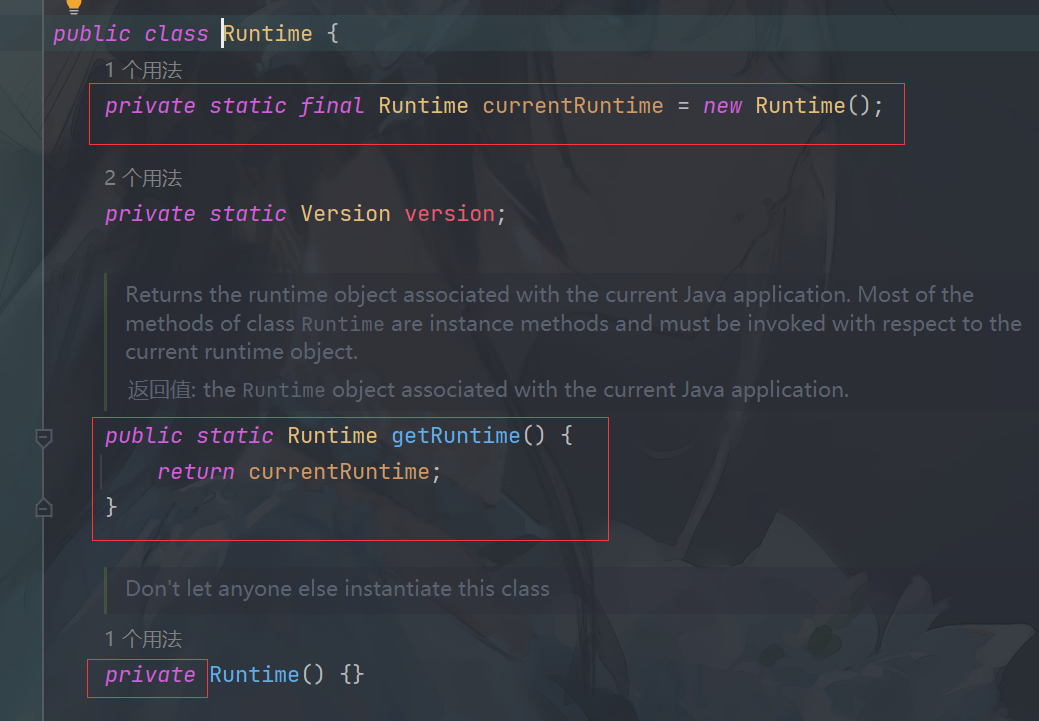java执行命令

``````Runtime run = Runtime.getRuntime();//获取 Runtime 对象
run.exec("calc");//执行calc命令弹出计算器``````

#### getMethod()``````Class clazz = Class.forName("java.lang.Runtime");
Method cmd = clazz.getMethod("exec", String.class);``````

#### invoke()

`invoke()` 方法用于执行 `getMethod` 获取的方法，看看它的相关参数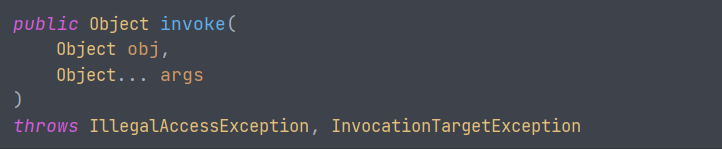``````Runtime run = Runtime.getRuntime();//获取Runtime对象
Class clazz = Class.forName("java.lang.Runtime");
Method cmd = clazz.getMethod("exec", String.class);
cmd.invoke(run, "calc");//执行函数``````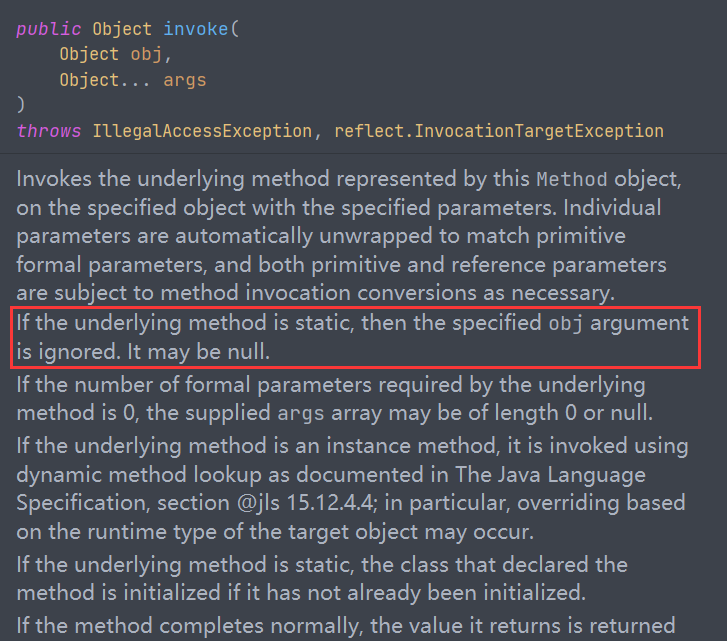``````Class clazz = Class.forName("java.lang.Runtime");
//调用静态方法getRuntime()获取Runtime对象
Runtime run = (Runtime) clazz.getMethod("getRuntime").invoke(null);//调用的是静态方法，invoke()里参数随便写一个就行，会忽略第一个参数
run.exec("calc");``````

#### 利用反射执行命令

`Object.Method(arg1, arg2, ...)` ，或执行静态方法 `Class.Method(arg1, arg2, ...)`

``````//获取 Runtime 类
Class clazz = Class.forName("java.lang.Runtime");
clazz.getMethod("exec",String.class).invoke(clazz.getMethod("getRuntime").invoke(null),
"calc");``````

``Class.forName("java.lang.Runtime").getMethod("exec", String.class).invoke(Class.forName("java.lang.Runtime").getMethod("getRuntime").invoke(null), "calc");//执行calc命令``

#### getConstructor和getDeclaredConstructor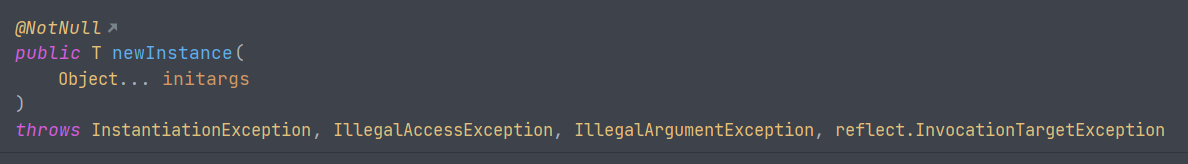`newInstance` 方法的参数是调用的构造方法对应的参数。

``new ProcessBuilder("calc").start();``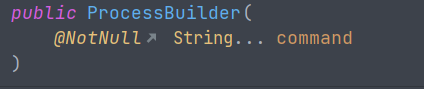`String... command` 等价于 `String[] command`

`````` Class clazz = Class.forName("java.lang.ProcessBuilder");
((ProcessBuilder) clazz.getConstructor(String[].class).newInstance(new String[][] {{"calc"}})).start();``````

``````Class clazz = Class.forName("java.lang.ProcessBuilder");
Constructor m = clazz.getConstructor(String[].class);
clazz.getMethod("start").invoke(m.newInstance(new String[][] {{"calc"}}));``````

``Class.forName("java.lang.ProcessBuilder").getMethod("start").invoke(Class.forName("java.lang.ProcessBuilder").getConstructor(String[].class).newInstance(new String[][] {{"calc"}}));``

### ProcessBuilder类探究

``Runtime.getRuntime().exec("calc");``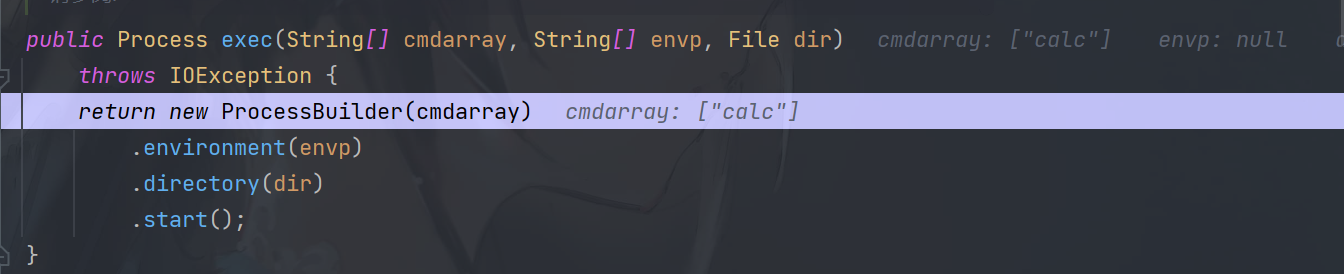``new ProcessBuilder("calc").start();``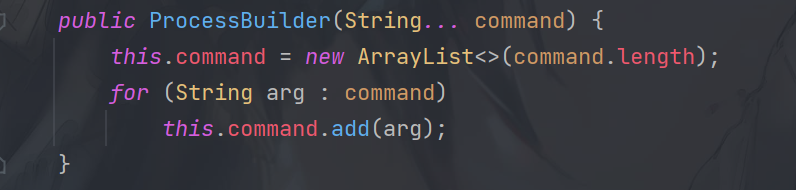`ProcessBuilder` 类中的一个构造方法参数是可变参数，即可以传递 `String[]` 类型

``Runtime.getRuntime().exec("calc");``

``new ProcessBuilder(new String[] {"calc"}).start();``